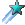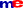# Is there an age problem

This Lesson (Solving Age Problems) was created by by algebrahouse.com(1657): View Source, Show
About algebrahouse.com: Visit: algebrahouse.com to ask questions and for notes, examples, and more.

When solving age problems, you need to represent the following in terms of a variable:
- the present ages of the people or things involved
- the age, at the other specified time, of the people or things involved

Then, form an equation based on these representations.

Here are some examples:

--------------------------------------------------------------------------------

Cary is 9 years older than Dan. In 7 years, the sum of their ages will equal 93.
Find both of their ages now.

x = Dan's age now
x + 9 = Cary's age now {Cary is 9 yrs older than Dan}

x + 7 = Dan's age in 7 years
x + 16 = Cary's age in 7 years

x + 7 + x + 16 = 93 {in seven years the sum of their ages will be 93}
2x + 23 = 93 {combined like terms}
2x = 70 {subtracted 23 from both sides}
x = 35 {divided both sides by 35}
x + 9 = 44 {substituted 35, in for x, into x + 9}

Dan is 35
Cary is 44

For more help from me, visit: www.algebrahouse.com

--------------------------------------------------------------------------------

Fred is 4 times as old as his niece, Selma. Ten years from now,
he will be twice as old as she will be. How old is each now?

x = Selma's age now
4x = Fred's age now {Fred is 4 times as old as Selma}

x + 10 = Selma's age in 10 years
4x + 10 = Fred's age in 10 years

4x + 10 = 2(x + 10) {his age in 10 yrs is twice her age in 10 years}
4x + 10 = 2x + 20 {used distributive property}
2x + 10 = 20 {subtracted 2x from both sides}
2x = 10 {subtracted 10 from both sides}
x = 5 {divided both sides by 2}
4x = 20 {substituted 5, in for x, into 4x}

Selma is 5 now
Fred is 20 now

For more help from me, visit: www.algebrahouse.com

--------------------------------------------------------------------------------

An eagle is 4 times as old as a falcon. Three years ago,
the eagle was 7 times as old as the falcon. Find the present
age of each bird.

x = falcon's age now
4x = eagle's age now {the eagle is 4 times as old as falcon}

x - 3 = falcon's age 3 years ago
4x - 3 = eagle's age 3 years ago

4x � 3 = 7(x � 3) {three years ago, eagle was 7 times the falcon}
4x � 3 = 7x � 21 {used distributive property}
4x = 7x -18 {added 3 to both sides}
-3x = -18 {subtracted 7x from both sides}
x = 6 {divided both sides by -3}
4x = 24 {substituted 6, in for x, into 4x}

falcon is 6 now
eagle is 24 now

For more help from me, visit: www.algebrahouse.com

--------------------------------------------------------------------------------

Brenda is 4 years older than Walter, and Carol is twice as old
as Brenda. Three years ago, the sum of their ages was 35.
How old is each now?

x = Walter's age now
x + 4 = Brenda's age now {Brenda is 4 yrs older than Walter}
2(x + 4) = 2x + 8 = Carol's age now {Carol is twice as old as Brenda, used distributive property}

x - 3 = Walter's age 3 years ago {subtracted 3 from x}
x + 1 = Brenda's age 3 years ago {subtracted 3 from x + 4}
2x + 5 = Carol's age 3 years ago {subtracted 3 from 2x + 8}

(x - 3) + (x + 1) + (2x + 5) = 35 {sum of ages, 3 years ago, was 35}
x - 3 + x + 1 + 2x + 5 = 35 {took out parentheses}
4x + 3 = 35 {combined like terms}
4x = 32 {subtracted 3 from both sides}
x = 8 = Walter now {divided both sides by 4}
x + 4 = 12 = Brenda now {substituted 8, in for x, into x + 4}
2(x + 4) = 24 = Carol now {substituted 8, in for x, into 2(x + 4)}

Walter is 8 now
Brenda is 12 now
Carol is 24 now

For more help from me, visit: www.algebrahouse.com

--------------------------------------------------------------------------------

This lesson has been accessed 251149 times.